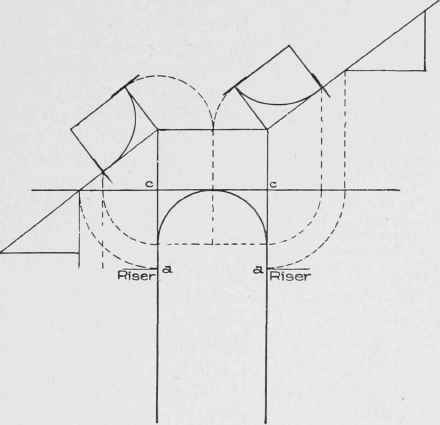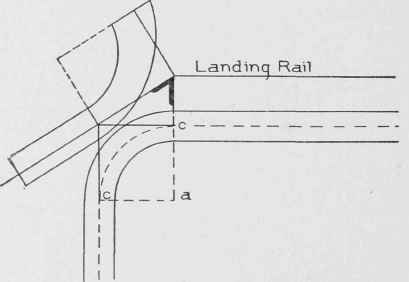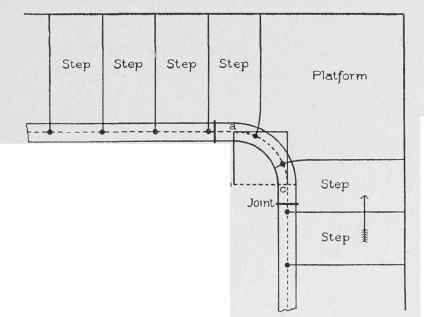It has been shown how to find the angle between the tangents of the face-mould, and that the angle is for the purpose of squaring the joints at the ends of the wreath. In Fig. 119 is shown how to lay out the curves by means of pins and a string - a very common practice among stair-build-ers. In this example the face-mould has equal tangents as shown at c" and d". The angle between the two tangents is shown at m as it will be required on the face-mould. In this figure a line is drawn from m parallel to the line drawn from h, which is marked in the diagram as "Directing Ordinate of Section." The line drawn from m will contain the minor axes; and a line drawn through the corner of the section at 3 will contain the major axes of the ellipses that will constitute the curves of the mould.

The major is to be drawn square to the minor, as shown. Place, from point 3, the circle shown on the minor, at the same distance as the circle in the plan is fixed from the point o. The diameter of this circle indicates the width of the curve at this point. The width at each end is determined by the bevels. The distance a b, as shown upon the long edge of the bevel, is equal to 1/2 the width of the mould, and is the hypotenuse of a right-angled triangle whose base is 1/2 the width of the rail. By placing this dimension on each side of n, as shown at b and 6, and on each side of h" on the other end of the mould, as shown also at b and b, we obtain the points b 2 b on the inside of the curve, and the points b 1 b on the outside. It will now be necessary to find the elliptical curves that will contain these points; and before this can be done, the exact length of the minor and major axes respectively must be determined. The length of the minor axis for the inside curve will be the distance shown from 3 to 2; and its length for the outside will be the distance shown from 3 to 1.Fig. 124. Arrangement of Risers Around Well-Hole with Rad ius Larger Than One-Half Width of Tread.Fig. 125. Arrangement of Risers around Well-Hole, with Risers Spaced Full Width of Tread.Fig. 126. Plan of Stair Shown in Fig. 123.Fig. 127. Plan of Stair Shown in Fig. 124.Fig. 128. Plan of Stair Shown in Fig. 125.

To find the length of the major axis for the inside, take the length of half the minor for the inside on the dividers: place one leg on b, extend to cut the major in z, continue to the minor as shown at k. The distance from b to k will be the length of the semi-major axis for the inside curve.

To draw the curve, the points or foci where the pins are to be fixed must be found on the major axis. To find these points, take the length of b k (which is, as previously found, the exact length of the semi-major for the inside curve) on the dividers; fix one leg at 2, and describe the arc Y, cutting the major where the pins are shown fixed, at o and o. Now take a piece of string long enough to form a loop around the two and extending, when tight, to 2, where the pencil is placed; and, keeping the string tight, sweep the curve from b to b.Fig. 129. Drawing Face-Mould for Wreath from Pitch-Board.Fig. 130. Development of Face-Mould for Wreath Connecting Rail of Flight with Level-Landing Rail.Fig. 131. Arranging Risers in Quarter-Turn between Two Flights.

The same method, for finding the major and foci for the outside curve, is shown in the diagram. The line drawn from b on the outside of the joint at n, to w, is the semi-major for the outside curve; and the points where the outside pins are shown on the major will be the foci. To draw the curves of the mould according to this method, which is a scientific one, may seem a complicated problem; but once it is understood, it becomes very simple. A simpler way to draw them, however, is shown in Fig. 120.Fig. 132. Arrangement of Risers around Quarter-Turn Giving Tangents Equal Pitch with Connecting Flight.

The width on the minor and at each end will have to be determined by the method just explained in connection with Fig. 119. In Fig 120, the points b at the ends, and the points in which the circumference of the circle cuts the minor axis, will be points contained in the curves, as already explained. Now take a flexible lath; bend it to touch b, z, and b for the inside curve, and b, w, and b for the outside curve. This method is handy where the curve is comparatively flat, as in the example here shown; but where the mould has a sharp curvature, as in case of the one shown in Fig. 101, the method shown in Fig. 119 must be adhered to.Fig. 133. Finding Bevel for Wreath of Plan, Fig. 132.Fig. 134. Well-Hole with Riser in Center. Tangents of Face-Mould, and Central Line of Rail, Developed.

With a clear knowledge of the above two methods, the student will be able to put curves on any mould.

The mould shown in these two diagrams, Figs. 119 and 120, is for the upper wreath, extending from h to n in Fig. 94 A practical handrailer would draw only what is shown in Fig. 120. He would take the lengths of tangents from Fig. 94, and place them as shown at h m and m n. By comparing Fig. 120 with the tangents of the upper wreath in Fig. 94, it will be easy for the student to understand the remaining lines shown in Fig. 120. The bevels are shown applied to the mould in Fig. 105, to give it the twist. In Fig. 106, is shown how, after the rail is twisted and placed in position over and above the quadrant c d in Fig. 94, its sides will be plumb.Fig. 135. Arrangement of Risers in Stair with Obtuse-Angle Plan.Fig. 136. Arrangement of Risers in Obtuse-Angle Plan, Giving Equal Pitch over Tangents and Flights. Face-Mould Developed.

In Fig. 121 are shown the tangents taken from the bottom wreath in Fig. 95 It was shown how to develop the section and find the angle for the tangents in the face-mould, m Fig. 113. The method shown in Fig. 119 for putting on the curves, would be the most suitable.

Fig. 121 is presented more for the purposes of study than as a method of construction. It, contains all the lines made use of to find the developed section of a plane inclining unequally in two different directions, as shown in Fig. 122.Fig. 137. Arrangement of Risers in Flight with Curve at Landing.